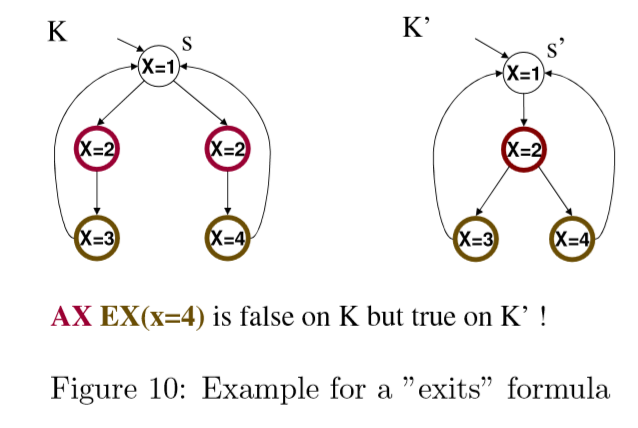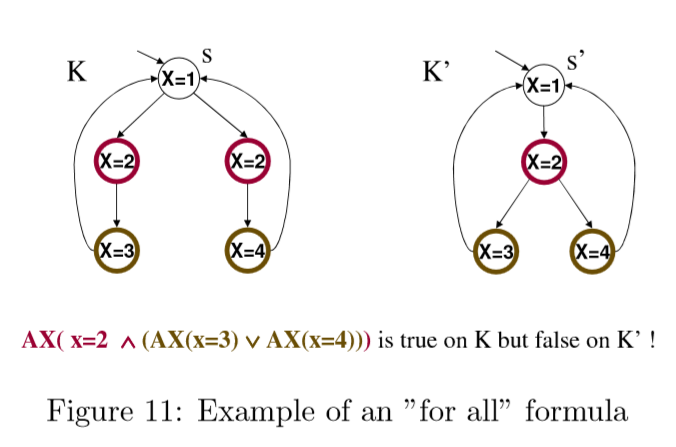==================AX是相对于初始节点（x=1）开始的树而言的，由于AX表示对于任何从初始节点(x=1)开始的路径中的下一个节点，因此EX则是相对于初始节点的子节点（x=2）开始的子树而言的。EX(x=4)表示存在从节点(x=2)开始的路径，使得子树的初始节点(x=2)的下一个节点是节点(x=4)。
在Kripke结构K中，对于(x=1) => (x=2) => (x=3) => (x=1)的路径而言(x=2)的下一个节点是(x=3)并不满足EX(x=4)，并不是对任何从初始节点(x=1)出发的路径都满足式子中的AX。因此K不满足式子AX EX(x=4)。相反K’中对于节点(x=2)而言，存在路径 (x=2) => (x=4) => (x=1) => (x=2)满足EX(x=4)，同时它也满足AX。因此K’满足。AX(x=3) V AX(x=4)要求在K’中，从(x=2)出发的任何路径，下一个节点都是(x=3)或者都是(x=4)。但是K’中(x=2)节点的下一个节点或是(x=3)或是(x=4),并不满足条件。Welcome！
I'm happy it's useful to you!
Show comments from Gitment